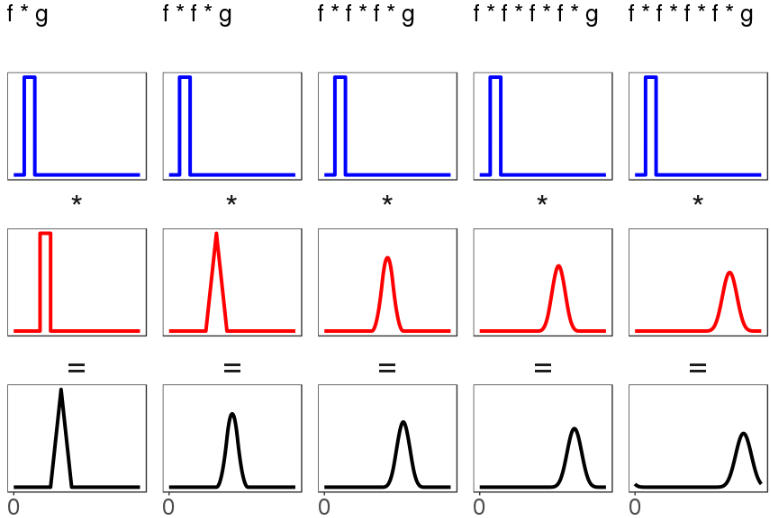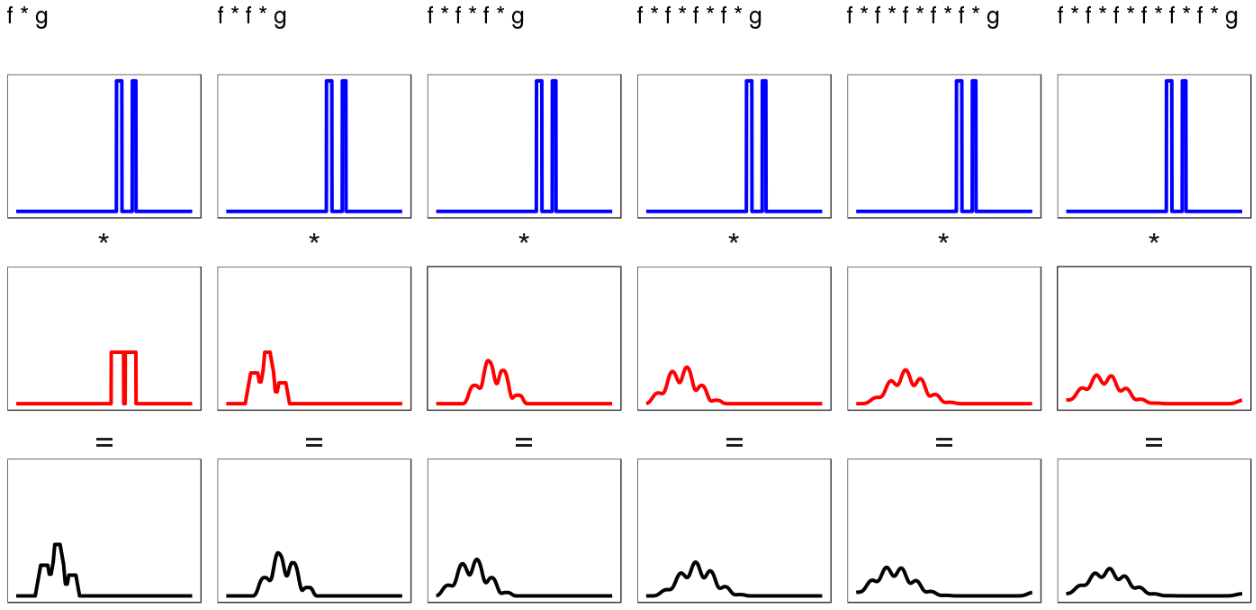27

Convolutions smooth out hard sharp things into nice smooth things. (See previous post for why convolutions are important). Here are some convolutions between various distributions:

(For all five examples, all the action in the functions  and  is in positive regions - i.e.  > 0 only when x > 0, and  too - though this isn't necessary.)

Things to notice:

• Everything is getting smoothed out! Even the bimodal case, in the column all the way to the right, has a convolution that looks like it's closer to something smooth than its two underlying distributions are. You might say the spike that uniform  uniform gives doesn't seem more smooth, exactly, than the uniform distributions that led to it. But notice the second column, which convolves a third uniform distribution with the result of the convolution from the first column. That second convolution leads to something more intuitively smooth (or if not smooth, at least more hill-shaped). We can repeat this procedure, feeding the output of the first convolution to the next, and each time we get a black result distribution that is a little bit shorter and a little bit wider. Thus we get a smooth thing by repeatedly convolving two input sharp things (f and g):The more we do this (for these particular shapes f and g), the more the final distribution will resemble a Gaussian. In other words, the central limit theorem applies to sets of uniform distributions.
• Even the more difficult bimodal distributions smooth out under repeated convolution, although it takes longer:I'm not sure whether this goes into a Gaussian or not. But the shape is suggestive.
•  (for positive  and ) is always to the right of  and  both. Because all these example  and  are positive, their means are positive. And it's a theorem that the mean  is always equal to the sum of the underlying means . So the location of the distributions is always increasing. Of course, for  and  with negative first moments, the location of  would go leftward; and if  had negative mean but  had positive mean, the mean of  could go anywhere. I am actually just describing how addition works, but the idea that you could locate a distribution  just by summing the locations of  and  was so shocking to me initially that I feel like I should emphasize it.

Understanding convolution as smoothing tells us about the central limit theorem

Convolution acts as a kind of "smearing" operator that people who use kernel density estimation across a variety of kernels will be familiar with; once you standardize the result... there's clear a progression toward increasingly symmetric hill shapes as you repeatedly smooth (and it doesn't much matter* if you change the kernel each time).

(a kernel is like a distribution; italics are mine.)

Up till now I've just called it "smoothing", but convolution on most (all?) probability distributions is better described as a smoothing-symmetricking-hillshaping operation. The graphics above are examples. And remember: The central limit theorem is a statement about convolutions on probability distributions! So here's a restatement of the basic central limit theorem:

Convolution on probability distributions is a smoothing-symmetricking-hillshaping operation, and under a variety of conditions, applying it repeatedly results in a symmetric hill that looks Gaussian.

Or you could look at it the other way around: The central limit theorem describes what comes out on the other side of repeated convolutions. If someone asked you what the behavior of  was, you could answer with the contents of the central limit theorem

Because your answer was the central limit theorem, it would contain information about: how varying the shapes of the component distributions  affected the shape of ; how many distributions  you needed to get close to some target distribution ; and what the  needed to look like in order for  to look Gaussian. It would also contain the same kind of information for the moments of , like the mean. And the variance. It would describe how to use the skew (which corresponds to the third moment) to evaluate how many distributions  you needed for  to look Gaussian (the Berry-Esseen theorem).

These information, together with various additional conditions and bounds, constitute a network of facts about what kinds of probability distributions  cause  to look Gaussian, and how Gaussian it will look. We call this network of facts "the central limit theorem", or sometimes - to emphasize the different varieties introduced by relaxing or strengthing some of the conditions - the central limit theorems.

*: While this kind of thing is true for many distributions, see Gurkenglas in the comments for why we shouldn't take "it doesn't much matter if you change the kernel each time" too literally.

27

Pingbacks
New Comment

and it doesn't much matter if you change the kernel each time

That's counterintuitive. Surely for every  there's an  that'll get you anywhere? If .

Yes, that sounds right - such an  exists. And expressing it in fourier series makes it clear. So the “not much” in “doesn’t much matter” is doing a lot of work.

I took his meaning as something like "reasonably small changes to the distributions  in don’t change the qualitative properties of ". I liked that he pointed it out, because a common version of the CLT stipulates that the random variables must be identically distributed, and I really want readers here to know: No! That isn’t necessary! The distributions can be different (as long as they’re not too different)!

But it sounds like you’re taking it more literally. Hm. Maybe I should edit that part a bit.

The fourier transform as a map between function spaces is continuous, one-to-one and maps gaussians to gaussians, so we can translate "convoluting nice distribution sequences tends towards gaussians" to "multiplying nice function sequences tends towards gaussians". The pointwise logarithm as a map between function spaces is continuous, one-to-one and maps gaussians to parabolas, so we can translate further to "nice function series tend towards parabolae", which sounds more almost always false than usually true.

In the second space, functions are continuous and vanish at infinity.
This doesn't work if the fourier transform of some function is somewhere negative, but then multiplying the function sequence has zeroes.
In the third space, functions are continuous and diverge down at infinity.

Hm. What you're saying sounds reasonable, and is an interesting way to look at it, but then I'm having trouble reconciling it with how widely the central limit theorem applies in practice. Is the difference just that the space of functions is much larger than the space of probability distributions people typically work with? For now I've added an asterisk telling readers to look down here for some caution on the kernel quote.

Suppose you take a bunch of differentiable functions, all of which have a global maximum at 0, and add them pointwise.

Usually you will get a single peak at 0 towering above the rest. The only special case is if  In the neighbourhood of 0, the function is approximately parabolic. (Its differentiable.) You take the exponent, this squashes everything but the highest peak down to nearly 0. (In relative terms). The highest peak turns into a sharp spiky Gaussian . You take the inverse Fourier transform and get a shallow Gaussian.

Even if you are unlucky enough to start with several equally high peaks in your 's then you still get something thats kind of a Gaussian. This is the case of a perfectly multimodal distribution, something 0 except on exact multiples of a number. The number of heads in a million coin flips forms a Gaussian out of dirac deltas at the integers.

But the condition of having a maximum at 0 in the Fourier transform is weaker than always being positive. If   then